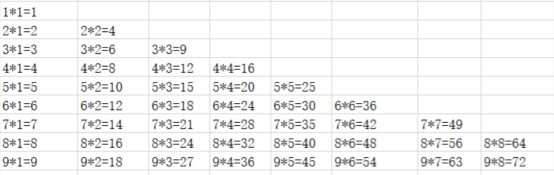# python如何将九九乘法表写入到Excel？```import xlwings as xw
import os
curpath=os.path.dirname(__file__)
filename=os.path.join(curpath,'example_99.xlsm')
@xw.sub
def py_99():
wb = xw.Book.caller()
wt = wb.sheets
for i in range(1,10):
for j in range(1,10):
if i>=j:
wt.range(i+10,j).value=str(i)+'*'+str(j)+'='+str(i*j)

if __name__ == "__main__":
xw.Book(filename).set_mock_caller()
py_99()```